# GRADE 5 – SAMPEL PAPER FINAL FMO 2021

Berikut ini adalah soal beserta kunci jawaban Fermat Mathematic Olympiad (FMO) 2021 grade 5 (Sc: Edukultur Indonesia)

A. Warm-up (4 points per question / No points deducted for wrong answers)

1. Which figure should be filled into the cell with the question mark?A)B)C)D)E)2. Father leaves home at 7:00 to take Lucy to school at the speed of 30km/h. He arrives at 7:20 then immediately goes back home. Because it was raining, he can only travel at two thirds or the original speed. When does he get back home?
A) 7:40
B) 7:45
C) 7:50
D) 7:55
E) 8.00

3. . Refer to the pattern below, how many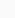are there in the 7th group?A) 36
B) 30
C) 24
D) 42
E) 18

4. The sum of three consecutive numbers is 111. Fill a number in the box with “?”.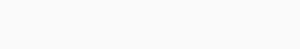A) 53
B) 27
C) 111
D) 31
E) 20

5. Find the value of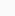given that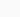is the greatest 2-digit number divisible by 6.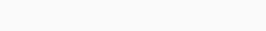A) 60
B) 61
C) 63
D) 65
E) 67

B. Speed-up (6 points per question / No points deducted for wrong answers)

6. Alex folds the square paper in half twice and cuts it as the figure below.       How many pieces does he receive?A) 4
B) 3
C) 12
D) 10
E) 5

7. Each letter represents a different digit. Find the sum of four digits A, G, E, S.A) 21
B) 22
C) 23
D) 24
D) 25

8. Given a $$4\times4$$ grid with each cell of side length 5cm. Find the area of the shaded region.A) $$40cm^2$$
B) $$210cm^2$$
C) $$180cm^2$$
D) $$150cm^2$$
E) $$200cm^2$$

9. Jamie has three times as many shirts as pants. Kellie has twice as many pants as shirts. They have 17 clothing items in total. How many shirts do they have altogether?
A) 9
B) 8
C) 7
D) 6
E) 5

10. A clothing store represents their sale in 4 weeks by the pie chart below. The sale in week 1 is the greatest and it reaches $1200. The sale in week 2 is equal to the sum of both week 3 and week 4. Find the sale in week 3, given that it is not the lowest.A)$900
B) $1000 C)$800
D) $600 E)$300

C. Challenge (8 points per question / No points deducted for wrong answers)

11. A, B, C, D and E are sitting on a bench. In which position is D sitting? Given that:
 A is sitting next to B to the right
 E is on the left end of the bench.
 C is sitting next to D but not A, E or B
A) Between C and A
B) Between C and B
C) Between A and E
D) Between E and C
E) Between A and E

12. Nine identical blocks of woods are combined to get the cube with surface area $$486cm^2$$. Find the surface area of one block of wood.A) $$81cm^2$$
B) $$54cm^2$$
C) $$126cm^2$$
D) $$163cm^2$$
E) None of the above

13. The shapes P and Q are formed from two and three identical rectangles,
respectively. Their perimeters are 48cm and 68cm respectively. What is the
perimeter of one small rectangle?A) 24cm
B) 28cm
C) 14cm
D) 26cm
E) 30cm

14. A box contains 3 red, 5 green, 4 blue, 6 yellow and 1 white balls. Suzy wants to get 3 balls with different colors. What is the minimum number of balls she should pick to be sure that she gets what she wants?
A) 11
B) 12
C) 4
D) 15
E) 16

15. The mobile balance below contains 5 Candy boxes and 1 Cookie box and one weight of 250g. Find the weight of a Cookie box.A) 200g
B) 100g
C) 300g
D) 50g
E) None of the above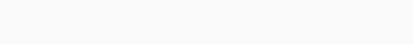How many truth-tellers are there in the line?